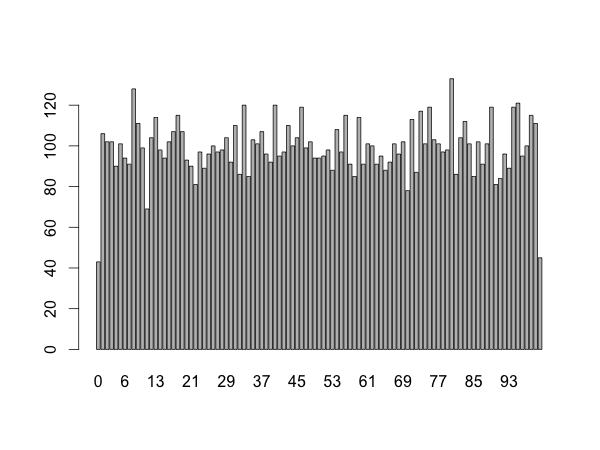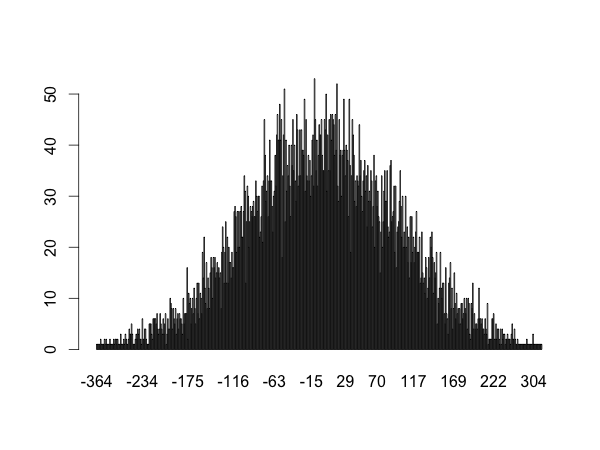Use source("filename.r") to run this.

```#utility functions

{
n <- readline(prompt="Enter an integer: ")
if(!grepl("^[0-9]+\$",n))
{
}
return(as.integer(n))
}

# real program start here

num <- round(runif(1) * 100, digits = 0)
guess <- -1

cat("Guess a number between 0 and 100.\n")

while(guess != num)
{
if (guess == num)
{
cat("Congratulations,", num, "is right.\n")
}
else if (guess < num)
{
cat("It's bigger!\n")
}
else if(guess > num)
{
cat("It's smaller!\n")
}
}
```
```> source("random-number-game.r")
Guess a number between 0 and 100.
Enter an integer: 50
It's smaller!
Enter an integer: 20
It's bigger!
Enter an integer: 40
It's bigger!
Enter an integer: 45
It's smaller!
Enter an integer: 43
It's bigger!
Enter an integer: 44
Congratulations, 44 is right.
```

The readinteger function has been explained in a previous example.

## Rounding

The round function rounds the first argument to the specified number of digits.

```> round(22.5,0)  # rounds to even number
 22
> round(3.14,1)
 3.1
```

## Getting a random number

runif generates random numbers between 0 and 1. The first argument specifies how many numbers you want.

```runif(2)
```
``` 0.8379240 0.1773677
```

The "unif" part of the function indicates that the numbers are uniformly distributed:This isn't completely uniform at the sides because of our use of round. A round to 0 only happens between 0 and 0.5 because we don't have negative numbers.

A non-uniform normal distribution would look like this:Learn how to make these graphs here.